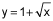Bobby P.

# Find the volume of the solid formed by rotating the region bounded by the graph of y=1 + √x , the y-axis, and the line y = 3 about the x-axis.

Find the volume of the solid formed by rotating the region bounded by the graph of, the y-axis, and the line y = 3 about the x-axis

8.376

41.888

26.667

none of these

## 2 Answers By Expert Tutors

By:Semi-Retired Mechanical Engineer & Mathematician